# 真空选型实例-计算

Z轴 ：5 m/s2

m = L x B x H x ρ

m = 重量[kg]

L = 长度[m]

B = 宽度[m]

H = 高度[m]

ρ = 密度[kg/m3]

m = 2.5 m x 1.25 m x 0.0025 m x 7,850 kg/m³

m = 61.33 kg

5 m/s²的加速度被提升 (无横向运动)。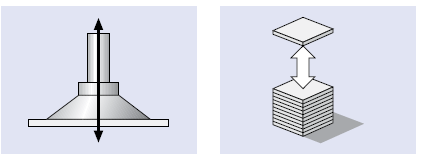FTH = m x (g + a) x S

FTH = 理论吸力[N]

m = 重量[kg]

g = 重力加速度[9.81 m/s2]

a = 系统加速度[m/s2]

S = 安全系数（最小值1.5，对于不均匀或透气材料以及

FTH = 61.33 kg x (9.81 m/s² + 5 m/s²) x 1.5

FTH = 1,363 N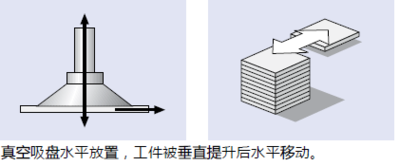FTH = m x (g + a / μ) x S

FTH = 理论吸力[N]

Fa = 加速度产生的力 = m · a

m = 重量[kg]

g = 重力加速度[9.81 m/s2]

a = 系统加速度 [m/s2] (包括“急停”情况！)

μ = 摩擦系数

= 0.1油性表面

= 0.2~0.3潮湿表面

= 0.5木材、金属、玻璃、石材表面

= 0.6粗糙表面

S = 安全系数 (最小值1.5，对于不均匀或透气材料以及

FTH = 61.33 kg x (9.81 m/s² + 5 m/s2 / 0.5) x 1.5

FTH = 1,822 N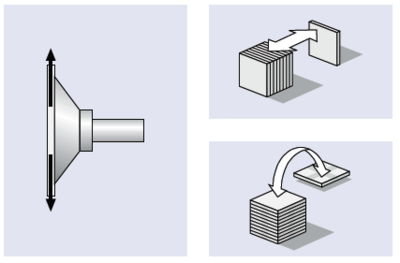FTH = (m /μ) x (g + a) x S

FTH = 理论吸力[N]

m = 重量[kg]

g = 重力加速度[9.81 m/s2]

a = 系统加速度 [m/s2] (包括“急停”情况)

μ = 摩擦系数

= 0.1油性表面

= 0.2~0.3潮湿表面

= 0.5木材、金属、玻璃、石材表面

= 0.6粗糙表面

S = 安全系数 (最小值2，对于不均匀或透气材料以及粗

FTH = (61.33 kg / 0.5) x (9.81 m/s2 + 5 m/s2) x 2

FTH = 3,633 N

1822 N。该理论吸持力为吸盘横向搬运工件所需的吸持力，可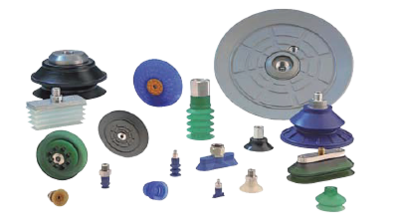6~8个吸盘。选择吸盘数量最重要的依据就是在搬运

FS = FTH / n

FS = 吸力

FTH = 理论吸力

n = 吸盘数量

FS = 304 N

FS = 1,822 N/8

FS = 228 N

6个PFYN-95-NBR吸盘

• 每个吸盘的实际吸力必须大于计算值。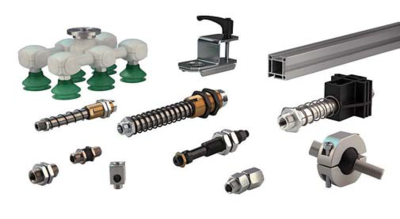（安装连接：G1/4内螺纹）

（连接至吸盘：G1/4外螺纹，缓冲行程75 mm）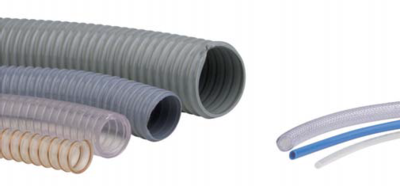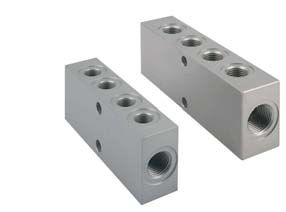VSL 8/6，外径8 mm，内径6 mm。用于连接6个真空吸盘。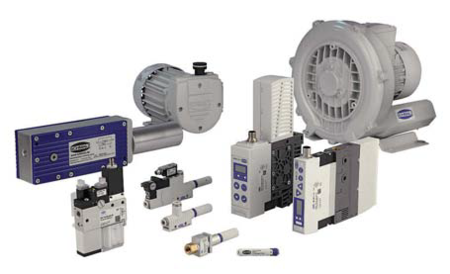不同吸盘直径下的抽吸速率 吸盘直径Ø 抽吸速率VS 抽吸速率VS 至60 mm 0.5 m3/h 8.3 l/min 至120 mm 1 m3/h 16.66 l/min 至215 mm 2 m3/h 33.3 l/min 至450 mm 4 m3/h 66.6 l/min

V = n x VS

n = 吸盘数量

VS = 单个吸盘所需的抽吸速率[m3/h, l/min]

V = 6 x 16.6 l/min

V = 99.6 l/min

0086-15623643088          E-mail:wecomes@wecomes.com.cn       地址：武汉市江夏区藏龙岛谭湖路8号工坊1栋2单元508室